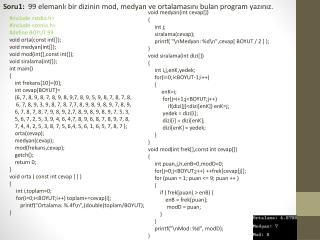# Soru1: 99 elemanlı bir dizinin mod , medyan ve ortalamasını bulan program yazınız. - PowerPoint PPT PresentationDownload PresentationSoru1: 99 elemanlı bir dizinin mod , medyan ve ortalamasını bulan program yazınız.

Soru1: 99 elemanlı bir dizinin mod , medyan ve ortalamasını bulan program yazınız.Download Presentation## Soru1: 99 elemanlı bir dizinin mod , medyan ve ortalamasını bulan program yazınız.

- - - - - - - - - - - - - - - - - - - - - - - - - - - E N D - - - - - - - - - - - - - - - - - - - - - - - - - - -
##### Presentation Transcript

1. Inference Probabilistic Graphical Models Variable Elimination Graph-Based Perspective

2. Initial Graph C C I I D D D D G G S S L L J J H H

3. Elimination as Graph Operation C • Eliminate: C I D D G S L J H Induced Markov network for the current set of factors

4. Elimination as Graph Operation C • Eliminate: D I D D G S L J H Induced Markov network for the current set of factors

5. Elimination as Graph Operation C • Eliminate: I I D G S L J H Induced Markov network for the current set of factors

6. Elimination as Graph Operation C • Eliminate: H I D G S L J H Induced Markov network for the current set of factors

7. Elimination as Graph Operation C • Eliminate: G I D G S L J H Induced Markov network for the current set of factors

8. Elimination as Graph Operation C • Eliminate: L,S I D G S L J H Induced Markov network for the current set of factors

9. Elimination as Graph Operation C • Eliminate: L,S I D G S L J H Induced Markov network for the current set of factors

10. Induced Graph C • The induced graph I, over factors  and ordering : • Undirected graph • Xi and Xj are connected if they appeared in the same factor in a run of the VE algorithm using  as the ordering I D D G S L J H

11. Cliques in the Induced Graph C • Theorem: Every factor produced during VE is a clique in the induced graph I D D G S L J H

12. Cliques in the Induced Graph C • Theorem: Every (maximal) clique in the induced graph is a factor produced during VE I D D G S L J H

13. Cliques in the Induced Graph C • Theorem: Every (maximal) clique in the induced graph is a factor produced during VE I D D G S L J H

14. Induced Width • The width of an induced graphis the number of nodes in the largest clique in the graph minus 1 • Minimal induced width of a graph K is min(width(IK,)) • Provides a lower bound on best performance of VE to a model factorizing over K

15. Finding Elimination Orderings • Theorem: For a graph H, determining whether there exists an elimination ordering for H with induced width  K is NP-complete • Note:This NP-hardness result is distinct from the NP-hardness result of inference • Even given the optimal ordering, inference may still be exponential

16. Finding Elimination Orderings • Greedy search using heuristic cost function • At each point, eliminate node with smallest cost • Possible cost functions: • min-neighbors: # neighbors in current graph • min-weight: weight (# values) of factor formed • min-fill: number of new fill edges • weighted min-fill: total weight of new fill edges (edge weight = product of weights of the 2 nodes)

17. Finding Elimination Orderings A • Theorem: The induced graph is triangulated • No loops of length > 3 without a “bridge” • Can find elimination ordering by finding a low-width triangulation of original graph H D B C

18. Square Root SAM, F. Dellaert and M. Kaess, IJRR, 2006 Robot Localization & Mapping

19. Robot Localization & Mapping ... x x x x x x 0 1 2 t 3 4 robot pose L1 z z z z z 1 2 t 3 4 L2 sensor observation L3

20. Square Root SAM, F. Dellaert and M. Kaess, IJRR, 2006 Robot Localization & Mapping

21. Square Root SAM, F. Dellaert and M. Kaess, IJRR, 2006 Eliminate Poses then Landmarks

22. Square Root SAM, F. Dellaert and M. Kaess, IJRR, 2006 Eliminate Landmarks then Poses

23. Square Root SAM, F. Dellaert and M. Kaess, IJRR, 2006 Min-Fill Elimination

24. Summary • Variable elimination can be viewed in terms of transformations on undirected graph • Eliminating Z connects its current neighbors • Sizes of cliques in resulting induced graph directly correspond to algorithm’s complexity • Keeping induced graph simple provides useful heuristics for selecting elimination ordering

25. END END END